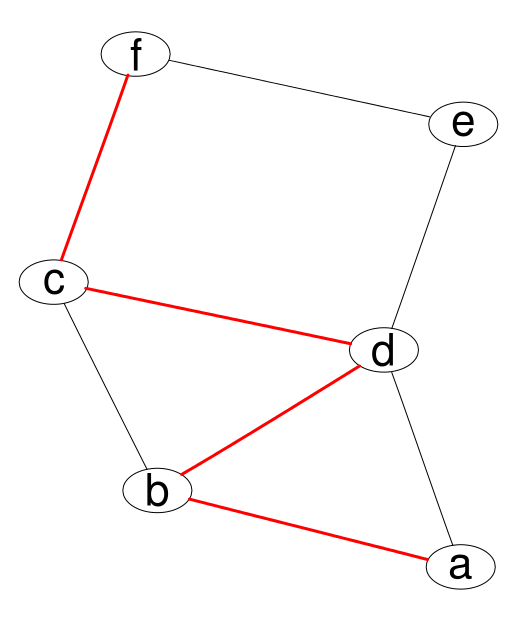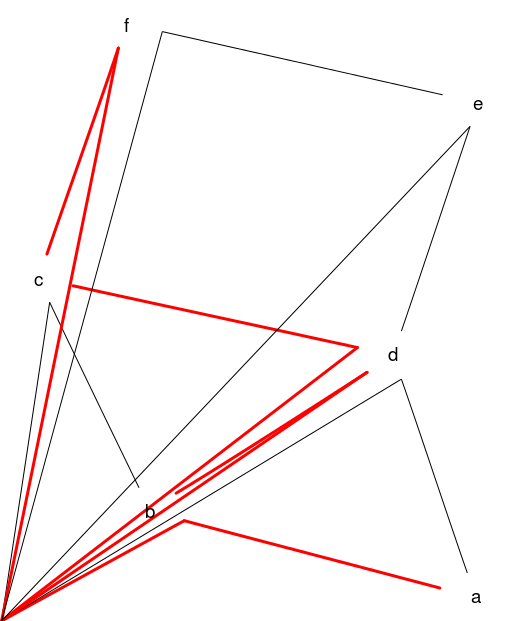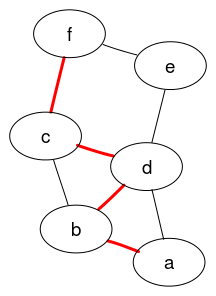# Example 5: showing a path

An undirected graph with 6 nodes, 8 edges, and a path between nodes marked out by red edges with line width of 3.

## Graph declaration

In all cases the graph is explicitly declared with ellipse shaped nodes. The dot file edges are declared in alphabetical order as this is the (assumed) default for how Rgraphviz will pass edges internally to graphviz.

 Dot file for graphviz: `````` graph { node [shape=ellipse]; //nodes a b c d e f; //edges a -- b[color=red,penwidth=3.0]; // red 3 width line along desired path b -- c; c -- d[color=red,penwidth=3.0]; d -- e; e -- f; a -- d; b -- d[color=red,penwidth=3.0]; c -- f[color=red,penwidth=3.0]; } `````` R code for Rgraphviz and gridGraphviz: ```# load Rgraphviz library library(Rgraphviz) # nodes nodes <- letters[1:6] # edges edgeList <- list(a=list(edges=c("b", "d")), b=list(edges=c("a", "c", "d")), c=list(edges=c("b", "d", "f")), d=list(edges=c("a", "b", "c", "e")), e=list(edges=c("d", "f")), f=list(edges=c("c", "e"))) graph <- new("graphNEL", nodes=nodes, edgeL=edgeList, edgemode="undirected") graph <- layoutGraph(graph) ```

## Graph rendering:

### gridGraphviz (new)

``````>> neato -Tpng ex5.dot -o ex5.png
`````````# Create Ragraph object
rag <- agopen(graph, "", layoutType="neato",
attrs=list(node=list(shape="ellipse")))

# mark out desired path
for (i in c(1, 4:6)) {
AgEdge(rag)[[i]]@lwd <- 3
AgEdge(rag)[[i]]@color <- "red"
}
```
```#plot
plot(rag)
``````library(gridGraphviz)

# Create Ragraph object
rag <- agopen(graph, "", layoutType="neato",
attrs=list(node=list(shape="ellipse")))

# mark out desired path
for (i in c(1, 4:6)) {
AgEdge(rag)[[i]]@lwd <- 3
AgEdge(rag)[[i]]@color <- "red"
}
```
```#plot
grid.graph(rag)
```
```## Warning: Unsupported node shape; using 'box'
## Warning: Unsupported node shape; using 'box'
## Warning: Unsupported node shape; using 'box'
## Warning: Unsupported node shape; using 'box'
## Warning: Unsupported node shape; using 'box'
## Warning: Unsupported node shape; using 'box'
``````library(gridGraphviz)

# Create Ragraph object
rag <- agopenTrue(graph, "", layoutType="neato",
attrs=list(node=list(shape="ellipse")))

# mark out desired path
for (i in c(1, 4:6)) {
AgEdge(rag)[[i]]@lwd <- 3
AgEdge(rag)[[i]]@color <- "red"
}
```
```#plot
grid.graph(rag)
```## Discussion

gridGraphviz (old) fails to produce ellipse shaped nodes, and produces "extra edges" which extend, in all cases, to the origin.